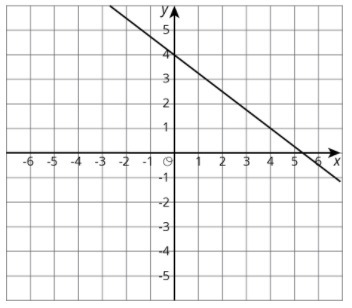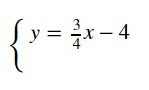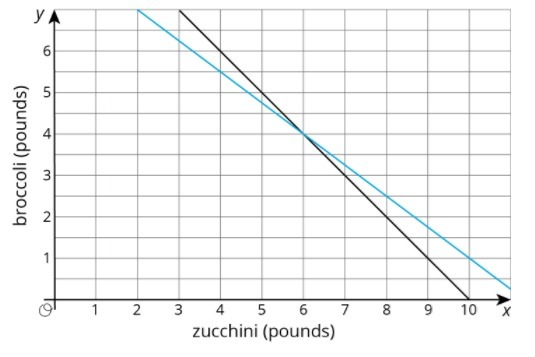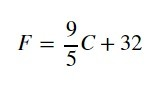PSABM5X2 - Practice---Acc7.5 Lesson 13 Systems of Equations (8.EE.C.8, 8.EE.C.8.a, 8.EE.C.8.b)
Part A)

Here is the graph for one equation in a system of equations:Write a second equation for the system so it has infinitely many solutions.

Complete the equation below

y = _______________

Part B)

Write a second equation whose graph goes through (0,1) so the system has no solutions.

Complete the equation below

y = _______________

Part C)

Write a second equation whose graph goes through (0,2) so the system has one solution at (4,1).

Complete the equation below

y = _______________

Create a second equation so the system has no solutions.Part A)

Andre is in charge of cooking broccoli and zucchini for a large group. He has to spend all $17 he has and can carry 10 pounds of veggies. Zucchini costs$1.50 per pound and broccoli costs $2 per pound. One graph shows combinations of zucchini and broccoli that weigh 10 pounds and the other shows combinations of zucchini and broccoli that cost$17.Name one combination of veggies that weighs 10 pounds but does not cost $17. Copied for free from openupresources.org Type your answer below: Part B) Name one combination of veggies that costs$17 but does not weigh 10 pounds.

Part C)

How many pounds each of zucchini and broccoli can Andre get so that he spends all $17 and gets 10 pounds of veggies? Zucchini: Type your answer below as a number (example: 5, 3.1, 4 1/2, or 3/2): Part D) How many pounds each of zucchini and broccoli can Andre get so that he spends all$17 and gets 10 pounds of veggies?

Broccoli:

Type your answer below as a number (example: 5, 3.1, 4 1/2, or 3/2):
Part A)

The temperature in degrees Fahrenheit, F, is related to the temperature in degrees Celsius, C, by the equationIn the Sahara desert, temperatures often reach 50 degrees Celsius. How many degrees Fahrenheit is this?

Type your answer below as a number (example: 5, 3.1, 4 1/2, or 3/2):
Part B)

In parts of Alaska, the temperatures can reach -60 degrees Fahrenheit. How many degrees Celsius is this?

Round to the nearest whole degree.

Type your answer below as a number (example: 5, 3.1, 4 1/2, or 3/2):
Part C)

There is one temperature where the degrees Fahrenheit and degrees Celsius are the same, so that C=F. Use the expression from the equation, where F is expressed in terms of C, to solve for this temperature.

Type your answer below as a number (example: 5, 3.1, 4 1/2, or 3/2):